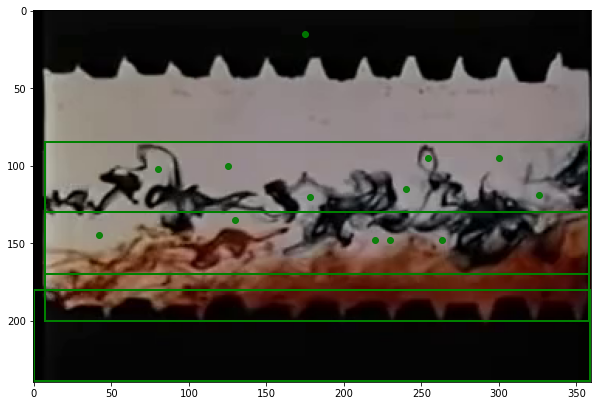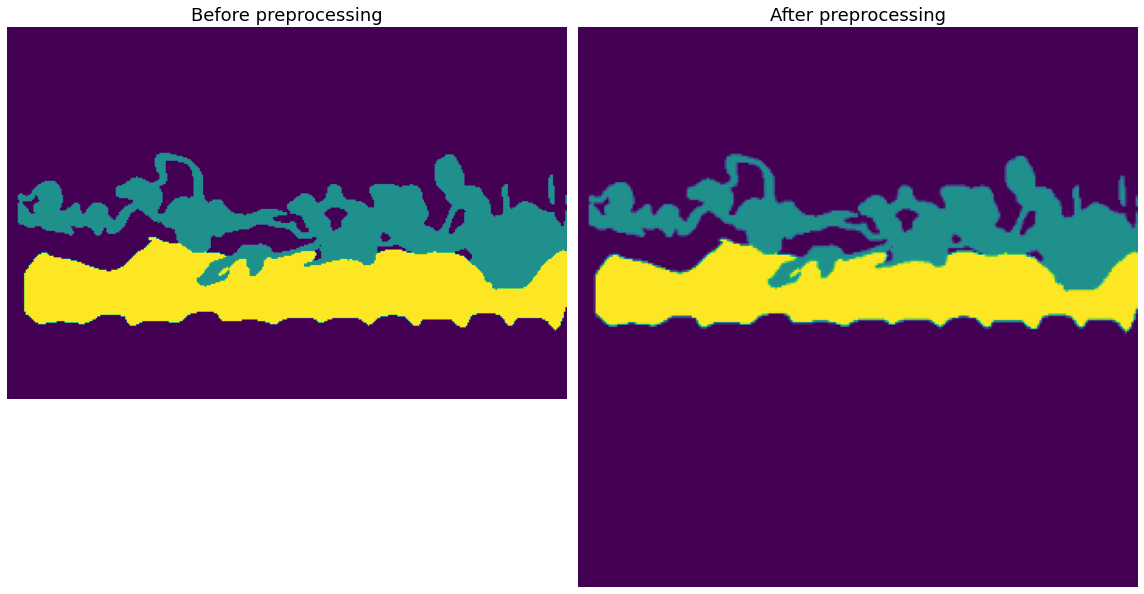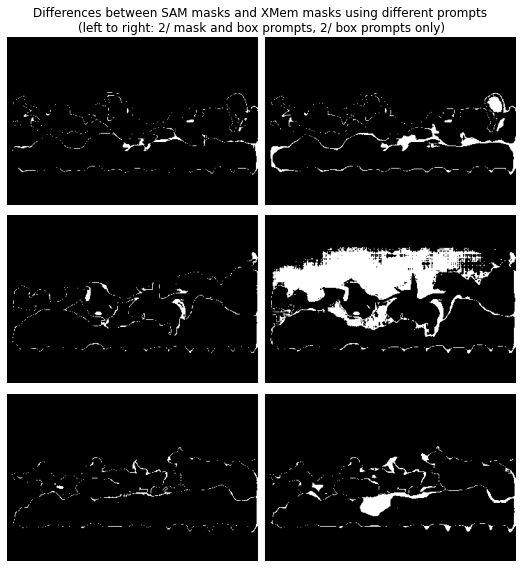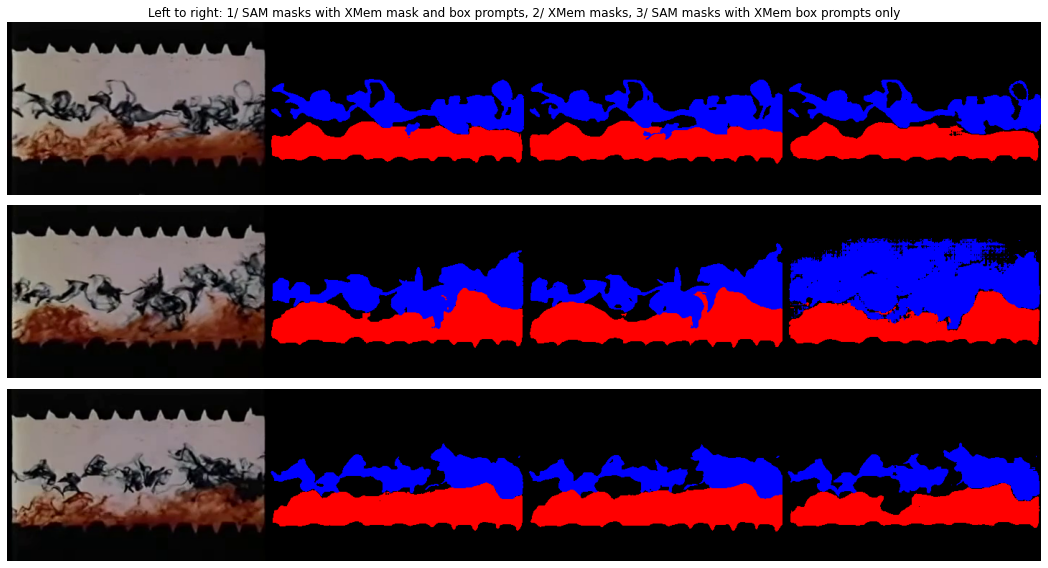## Contents

Here is a simple example demonstrating the use of Meta’s Segment Anything Model(SAM) on a video of turbulent fluid flow. The video is a clip from National Committee for Fluid Mechanics Films (NCFMF) collection video “Turbulence”. The clip shows the movement of two dyes moving through a channel that help to visualise turbulent flow. The dyes don’t mix much so throughout there are two fairly distinct areas of different colours but each one varys considerably in form across the video.

The utility of SAM is that it can be used to segment videos without ground truth masks. My approach was to segment the initial frame using SAM and then use XMem to propagate the segmentation to the rest of the video.

A notebook with the code for this example is available here.

## Data

These are the steps I followed to clip this section of the video:

yt-dlp https://www.youtube.com/watch?v=1_oyqLOqwnI

• Convert to mp4 using ffmpeg
ffmpeg -i <name of video>.webm <name of video>.mp4

n = 11
start = (13, 21)
end = (14, 2)
clip = video.subclip(start * 60 + start, end * 60 + end)
clip.write_videofile('fluids_{}_{:02d}{:02d}_{:02d}{:02d}.mp4'.format(n, *start, *end),
codec='libx264',
audio_codec='aac',
temp_audiofile='temp-audio.m4a',
remove_temp=True
)


## Segmentation using prompts

SAM lets you provide prompts as input to the model. The model then uses these prompts to segment images. The prompts can be:

• Points with foreground/background labels
• Bounding boxes

Since there are no ground truth masks for this video, I initially tried the first two options.

After a few experiments in which foreground points did not yield good results, I decided to use bounding boxes. However I also provided background points to help the model distinguish between the fluid and the background.

As you can see in the video, the fluid has loops and swirls so there are spaces in between that should be considered as background. The fluid is enclosed in a box with ridged surfaces to encourage turbulent flow and these should also be considered as background.

The top surface is remains clear of the fluid at all times and is straightforward to segment but the bottom surface is in contact with the fluid and it is not always easy to distinguish between the two. It did not suffice to provide background points for the bottom surface since sometimes it would end up getting segmented as part of the bottom fluid layer. Therefore I provided an additional bounding box for the bottom surface.## Running the model

You set up the predictor as follows:

import sys
sys.path.append("..")
from segment_anything import sam_model_registry, SamPredictor

sam_checkpoint = "/data/sam_vit_h_4b8939.pth"
model_type = "vit_h"

device = "cuda"

sam = sam_model_registry[model_type](checkpoint=sam_checkpoint)
sam.to(device=device)

predictor = SamPredictor(sam)


To generate masks, you first need to set the image for the predictor and then call one of its prediction methods. Here I am using the predict_torch method which lets you predict masks for multiple objects in a single image.

predictor.set_image(image)

input_boxes = torch.from_numpy(boxes).to(predictor.device)
transformed_boxes = predictor.transform.apply_boxes_torch(input_boxes, image.shape[:2])
# Comment the following to use background points
point_coords=None,
point_labels=None,
# Uncomment the following to use background points
# point_coords=torch.from_numpy(np.concatenate([bkgd])[None]).repeat(2, 1, 1).to(predictor.device),
# point_labels=torch.cat([torch.zeros(len(bkgd))])[None].repeat(2, 1).to(predictor.device),
boxes=transformed_boxes,
multimask_output=False, # return a single prediction per batch item
)


The output masks has shape [B, C, H, W] where C is the number of masks which will be 1 if multimask_output is False.

## Post-processing

The returned masks are post-processed as follows:

• Hole filling is applied to the raw logits
• The masks are merged by taking the argmax of the logits
• The bottom surface label is set to background
def masks2ann(raw_masks, alpha=None):

colours = np.stack(
[[0, 0, 0],
[0, 0, 255],
[255, 0, 0]]
)

if alpha is not None:
assert 0 <= alpha <= 1
colours = np.concatenate([colours, np.ones([len(colours), 1]) * alpha * 255], 1)

colours = colours.astype('uint8')

# +1 since 0 is background, then set pixels where no mask is greater than threshold to 0

# Set background to 0
merged = merged * (merged < 3)

return merged, colours[merged]I tried segmenting with and without the background points. Using background points leads to more artifacts and undesired holes that were not entirely eliminated by the post-processing step. So I decided to use the mask generated without background points downstream.

## XMem to propagate segmentation

The model requires you to provide the video as a sequence of JPEG images. An annotation is required only for the first frame. The annotation can contain multiple classes. Then we run this command from the XMem directory:

python eval.py --output /data/sam-xmem-fluids/outputs --dataset G --generic_path /data/sam-xmem-fluids/ --split val


The output is a sequence of PNG images with the same name as the input JPEG images. The PNG images contain the segmentation masks for each class.

As you can see the segmentation is reasonable initially but by about halfway the segmentation for the bottom layer has started to overwhelm the segmentation for the top layer, particularly towards the right side of the image. However the segmentation for the top layer is still quite good on the left side of the image.

In addition to box and prompt inputs, SAM also supports mask inputs. However the masks need to be in the same format as the raw non-resized logits output by the mask decoded. Here is an overview of the preprocessing and postprocessing steps that occur in the model.

Images are first resized so that the longest side is 1024x1024 and its dimensions permutation and expanded so that it has dimensions BxCxHxW

input_image = self.transform.apply_image(image)
input_image_torch = torch.as_tensor(input_image, device=self.device)
input_image_torch = input_image_torch.permute(2, 0, 1).contiguous()[None, :, :, :]


where

self.transform = ResizeLongestSide(sam_model.image_encoder.img_size)


and predictor.model.image_encoder.img_size is 1024

Then they are then padded to be 1024x1024

input_image = self.model.preprocess(transformed_image)


where preprocess is defined as

def preprocess(self, x: torch.Tensor) -> torch.Tensor:
"""Normalize pixel values and pad to a square input."""
# Normalize colors
x = (x - self.pixel_mean) / self.pixel_std

h, w = x.shape[-2:]
return x


The logit masks are of size 256x256 and to get the final masks they are first resized to 1024x1024

masks = F.interpolate(
(self.image_encoder.img_size, self.image_encoder.img_size),
mode="bilinear",
align_corners=False,
)


and then they are cropped to size of the image after the longest size has been resized to 1024 but before it has been padded to 1024x1024 i.e. after ResizeLongestSide but before preprocess and resized to the original image dimensions

def postprocess_masks(
self,
input_size: Tuple[int, ...],
original_size: Tuple[int, ...],
) -> torch.Tensor:
"""
Arguments:
in BxCxHxW format.
input_size (tuple(int, int)): The size of the image input to the
model, in (H, W) format. Used to remove padding.
original_size (tuple(int, int)): The original size of the image
before resizing for input to the model, in (H, W) format.
Returns:
(torch.Tensor): Batched masks in BxCxHxW format, where (H, W)
is given by original_size.
"""
(self.image_encoder.img_size, self.image_encoder.img_size),
mode="bilinear",
align_corners=False,
)


The masks for mask_input must have dimensions 256x256. Since we start off with masks with the same dimensions as the image, we should reverse postprocess_masks to get tensors of shape 256x256 which are in the right format. We can make use of the functions used to resize the image.

def preprocess_masks(
mask_input_size: Tuple[int, ...] = (256, 256),
) -> torch.Tensor:



It is evident that aspect ratio is preserved over the course of resizing.A further subtlety is that the masks should have a suitable range of values. The binary masks are generated by thresholding values above 0 but the logit masks output from the network are not bounded between 0 and 1. If you input binary masks the model ends up predicting background for everything. Here we have decided to map values > 0 to 18 and == 0 to -18 after looking at the values of the mask outputs and this yields reasonable results.

## SAM to propagate segmentation

Since the image only changes slightly from frame to frame, we can also try using SAM to propagate the segmentation as follows. We start off as before with a mask for the first frame generated using points and bounding boxes.

Then we get bounding boxes from the mask to use as prompts. Here is a simple function to do this:

def mask2box(mask):
any_x = non_zero.any(0)
x0 = np.argmax(any_x)

any_y = non_zero.any(1)

y0 = np.argmax(any_y)

return np.stack([x0, y0, x1, y1])


We also use the masks output by SAM as prompts. For the first frame we will use the initial mask to refine the segmentation whilst for subsequent frames the segmentation of the previous frame will be used to generate the segmentation.

vidcap = cv2.VideoCapture('fluids_11_1321_1402.mp4')
itr = 0

result = []

max_frames = int(vidcap.get(cv2.CAP_PROP_FRAME_COUNT))

# 1,2 for the foreground, 3 for background
max_label = 4

for itr in tqdm.trange(max_frames):

image_itr = cv2.cvtColor(image_itr, cv2.COLOR_BGR2RGB)
predictor.set_image(image_itr)

if itr == 0:
point_coords=None,
point_labels=None,
boxes=transformed_boxes,
return_logits=True
)

bounding_boxes = torch.from_numpy(bounding_boxes).to(predictor.device)
bounding_boxes = predictor.transform.apply_boxes_torch(bounding_boxes, image_itr.shape[:2])

m = torch.cat(
for i in range(1, max_label)
]
)

m[m > 0] = 18
m[m == 0] = -18

point_coords=None,
point_labels=None,
boxes=bounding_boxes,
return_logits=True,
)



The results are reasonable for about the first 9-10 seconds but thereafter the segmentation for the top layer floods the top half covering the background. The mask for the bottom layer remains more stable however.

## SAM to refine segmentation

Let us also try using SAM to refine the masks generated by XMem. The steps are similar except that for each frame we use the mask generated the by XMem rather than the mask generated by SAM in the previous frame.

mask_xmem_itr = cv2.imread(frames[itr])[..., -1]


I also tried using only bounding boxes generated from the XMem masks to see if this would yield better masks in cases where XMem fails.

The differences are fairly small between the masks generated by SAM using XMem masks as well as boxes as prompts compared to the masks generated by XMem.On the other hand using bounding boxes only from XMem masks as prompts is less stable sometimes yielding much better masks and sometimes much worse masks.